xDissociation (chemistry)Encyclopedia
Dissociation in chemistry and biochemistry
Biochemistry
Biochemistry, sometimes called biological chemistry, is the study of chemical processes in living organisms, including, but not limited to, living matter. Biochemistry governs all living organisms and living processes...

is a general process in which ionic compounds (complexes
Complex (chemistry)
In chemistry, a coordination complex or metal complex, is an atom or ion , bonded to a surrounding array of molecules or anions, that are in turn known as ligands or complexing agents...

, or salts) separate or split into smaller particles, ions, or radicals
Radicals are atoms, molecules, or ions with unpaired electrons on an open shell configuration. Free radicals may have positive, negative, or zero charge...

, usually in a reversible manner. For instance, when a Bronsted-Lowry acid
Acid
An acid is a substance which reacts with a base. Commonly, acids can be identified as tasting sour, reacting with metals such as calcium, and bases like sodium carbonate. Aqueous acids have a pH of less than 7, where an acid of lower pH is typically stronger, and turn blue litmus paper red...

is put in water, a covalent bond between an electronegative atom and a hydrogen atom is broken by heterolytic fission, which gives a proton and a negative ion
Ion
An ion is an atom or molecule in which the total number of electrons is not equal to the total number of protons, giving it a net positive or negative electrical charge. The name was given by physicist Michael Faraday for the substances that allow a current to pass between electrodes in a...

. Dissociation is the opposite of association and recombination. The process is frequently confused with ionization
Ionization
Ionization is the process of converting an atom or molecule into an ion by adding or removing charged particles such as electrons or other ions. This is often confused with dissociation. A substance may dissociate without necessarily producing ions. As an example, the molecules of table sugar...

.

## Dissociation constant

For reversible dissociations in a chemical equilibrium
Chemical equilibrium
In a chemical reaction, chemical equilibrium is the state in which the concentrations of the reactants and products have not yet changed with time. It occurs only in reversible reactions, and not in irreversible reactions. Usually, this state results when the forward reaction proceeds at the same...

AB A + B

the dissociation constant
Dissociation constant
In chemistry, biochemistry, and pharmacology, a dissociation constant is a specific type of equilibrium constant that measures the propensity of a larger object to separate reversibly into smaller components, as when a complex falls apart into its component molecules, or when a salt splits up into...

Kd is the ratio of dissociated to undissociated compound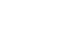where the brackets denote the equilibrium concentrations of the species.

## Dissociation degree

The dissociation degree is the fraction of original solute molecules that have dissociated. It is usually indicated by the Greek symbol. There is a simple relationship between this parameter and the van 't Hoff factor
Van 't Hoff factor
The van 't Hoff factor i is a measure of the effect of a solute upon colligative properties, such as vapor pressure, osmotic pressure and freezing point depression. The van 't Hoff factor is the ratio between the actual concentration of particles produced when the substance is dissolved, and the...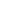. If the solute substance dissociates intoions, then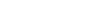For instance, for the following dissociation
KCl K+ + Cl-

As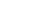, we would have that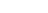## Salts

The dissociation of salts by solvation
Solvation
Solvation, also sometimes called dissolution, is the process of attraction and association of molecules of a solvent with molecules or ions of a solute...

in a solution
Solution
In chemistry, a solution is a homogeneous mixture composed of only one phase. In such a mixture, a solute is dissolved in another substance, known as a solvent. The solvent does the dissolving.- Types of solutions :...

like water means the separation of the anions and cations. The salt can be recovered by evaporation
Evaporation
Evaporation is a type of vaporization of a liquid that occurs only on the surface of a liquid. The other type of vaporization is boiling, which, instead, occurs on the entire mass of the liquid....

Solubility equilibrium
Solubility equilibrium is a type of dynamic equilibrium. It exists when a chemical compound in the solid state is in chemical equilibrium with a solution of that compound. The solid may dissolve unchanged, with dissociation or with chemical reaction with another constituent of the solvent, such as...

An electrolyte refers to a substance that contains free ions and can be used as an electrically conductive medium. Most of the solute does not dissociate in a weak electrolyte whereas in a strong electrolyte a higher ratio of solute dissociates to form free ions.

A weak electrolyte is a substance whose solute exists in solution mostly in the form of molecules, with only a small fraction in the form of ions. Simply because a substance does not readily dissolve does not make it a weak electrolyte. Acetic acid
Acetic acid
Acetic acid is an organic compound with the chemical formula CH3CO2H . It is a colourless liquid that when undiluted is also called glacial acetic acid. Acetic acid is the main component of vinegar , and has a distinctive sour taste and pungent smell...

(CH3COOH) and ammonium
Ammonium
The ammonium cation is a positively charged polyatomic cation with the chemical formula NH. It is formed by the protonation of ammonia...

(NH4+) are good examples. Acetic acid is extremely soluble in water, but most of the compound dissolves into molecules, rendering it a weak electrolyte. Weak bases and weak acids are generally weak electrolytes. In an aqueous solution there will be some CH3COOH and some CH3COO- and H+.

A Strong electrolyte is a solute that exists in solution completely or nearly completely as ions. Again, the strength of an electrolyte is defined as the percentage of solute that is ions, rather than molecules. The higher the percentage, the stronger the electrolyte. Thus, even if a substance is not very soluble, but what does dissolve dissociates completely into ions, the substance is defined as a strong electrolyte. Similar logic applies to a weak electrolyte. Strong acids and bases are good examples such as HCl, H2SO4, NaCl. These will all exist as ions in an aqueous media.

## Gases

The degree of dissociation in gases is denoted by the symbol α where α refers to the percentage of gas molecules which dissociate. Various relationships between Kp and α exist depending on the stoichiometry of the equation. The example of dinitrogen tetroxide
Dinitrogen tetroxide
Dinitrogen tetroxide is the chemical compound N2O4. It is a useful reagent in chemical synthesis. It forms an equilibrium mixture with nitrogen dioxide; some call this mixture dinitrogen tetroxide, while some call it nitrogen dioxide.Dinitrogen tetroxide is a powerful oxidizer, making it highly...

(N2O4) dissociating to nitrogen dioxide
Nitrogen dioxide
Nitrogen dioxide is the chemical compound with the formula it is one of several nitrogen oxides. is an intermediate in the industrial synthesis of nitric acid, millions of tons of which are produced each year. This reddish-brown toxic gas has a characteristic sharp, biting odor and is a prominent...

(NO2) will be taken.
N2O4 2NO2

If the initial concentration of dinitrogen tetroxide is 1 mole per litre, this will decrease by α at equilibrium giving, by stoichiometry, 2α moles of NO2. The equilibrium constant (in terms of pressure) is given by the equation;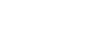Where p represents the partial pressure. Hence, through the definition of partial pressure
Partial pressure
In a mixture of ideal gases, each gas has a partial pressure which is the pressure which the gas would have if it alone occupied the volume. The total pressure of a gas mixture is the sum of the partial pressures of each individual gas in the mixture....

and using pT to represent the total pressure and x to represent the mole fraction;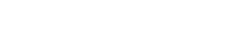The total number of moles at equilibrium is (1-α)+(2α) which is equivalent to 1+α. Thus, substituting the mole fractions with actual values in term of alpha and simplifying;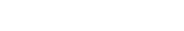This equation is in accordance with Le Chatelier's Principle. Kp will remain constant with temperature. The addition of pressure to the system will increase the value of pT so α must decrease to keep Kp constant. In fact, increasing the pressure of the equilibrium favours a shift to the left favouring the formation of dinitrogen tetroxide (as on this side of the equilibrium there is less pressure since pressure is proportional to number of moles) hence decreasing the extent of dissociation α.

## Acids

The dissociation of acids in a solution means the split-off of a proton
Proton
The proton is a subatomic particle with the symbol or and a positive electric charge of 1 elementary charge. One or more protons are present in the nucleus of each atom, along with neutrons. The number of protons in each atom is its atomic number....

H+, see Acid-base reaction theories
Acid-base reaction theories
An acid–base reaction is a chemical reaction that occurs between an acid and a base. Several concepts that provide alternative definitions for the reaction mechanisms involved and their application in solving related problems exist...

. This is an equilibrium process, meaning that dissociation and recombination takes place at the same time. The acid dissociation constant
Acid dissociation constant
An acid dissociation constant, Ka, is a quantitative measure of the strength of an acid in solution. It is the equilibrium constant for a chemical reaction known as dissociation in the context of acid-base reactions...

Ka is an indicator of the acid strength: stronger acids have a higher Ka value (and a lower pKa value).

## Fragmentation

Fragmentation
Fragmentation (chemistry)
Fragmentation is a type of chemical dissociation. Fragmentation of a molecule can take place by a process of heterolysis or homolysis.It is a phenomenon observed in mass spectrometry where it is used as a tool to find the structural formula of a molecule, process called structural elucidation.It...

of a molecule can take place by a process of heterolysis
Heterolysis
In chemistry, heterolysis or heterolytic fission is chemical bond cleavage of a neutral molecule generating a cation and an anion. In this process the two electrons that make up the bond are assigned to the same fragment...

or homolysis
Homolysis
In general it means breakdown to equal pieces There are separate meanings for the word in chemistry and biology.-Homolysis in chemistry:...

## Receptors

Receptors
Receptor (biochemistry)
In biochemistry, a receptor is a molecule found on the surface of a cell, which receives specific chemical signals from neighbouring cells or the wider environment within an organism...

are protein
Protein
Proteins are biochemical compounds consisting of one or more polypeptides typically folded into a globular or fibrous form, facilitating a biological function. A polypeptide is a single linear polymer chain of amino acids bonded together by peptide bonds between the carboxyl and amino groups of...

s that bind small ligands. The dissociation constant Kd is used as indicator of the affinity of the ligand to the receptor. The higher the affinity of the ligand for the receptor the lower the Kd value (and the higher the pKd value).

• Ionization
Ionization
Ionization is the process of converting an atom or molecule into an ion by adding or removing charged particles such as electrons or other ions. This is often confused with dissociation. A substance may dissociate without necessarily producing ions. As an example, the molecules of table sugar...

• Acid
Acid
An acid is a substance which reacts with a base. Commonly, acids can be identified as tasting sour, reacting with metals such as calcium, and bases like sodium carbonate. Aqueous acids have a pH of less than 7, where an acid of lower pH is typically stronger, and turn blue litmus paper red...

• Base (chemistry)
Base (chemistry)
For the term in genetics, see base A base in chemistry is a substance that can accept hydrogen ions or more generally, donate electron pairs. A soluble base is referred to as an alkali if it contains and releases hydroxide ions quantitatively...

• Thermal decomposition
Thermal decomposition
Thermal decomposition, or thermolysis, is a chemical decomposition caused by heat. The decomposition temperature of a substance is the temperature at which the substance chemically decomposes....